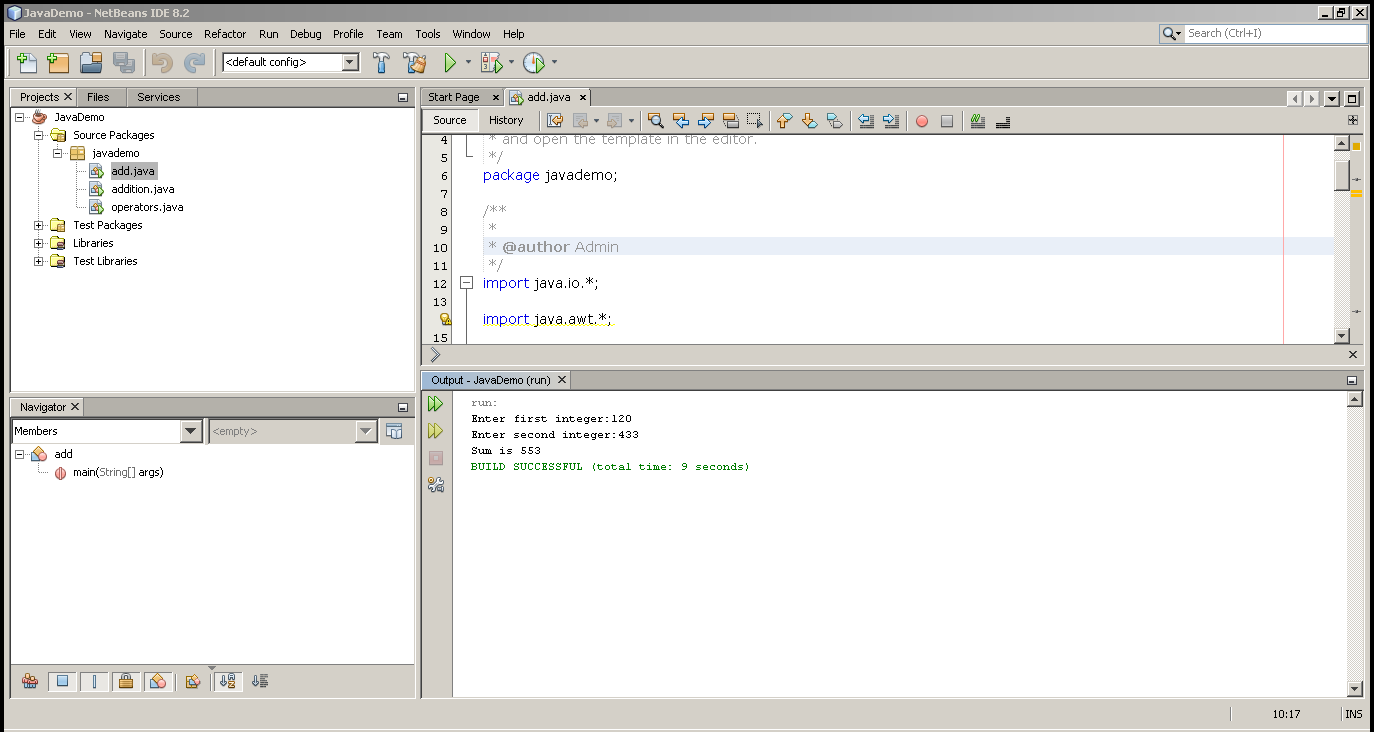## Program to add two numbers in Java

This is a simple program to demonstrate and learn the basics of Java programming. The Java program uses arithmetic operators and this is an example of the addition operator.

This program is written using JDK 8u111 with Netbeans IDE 8.2 on a Windows 7 64-bit PC. Alternatively, you can also compile and run this program from Windows Command line with only JDK installed.

## Problem Definition

In this program, we receive two input numbers from the user and add them using the addition (+) operator and display the sum.

## FlowchartFlowchart – Java Program to Add Two Numbers

## Program Code

``````import java.io.*;

import java.awt.*;

import javax.swing.*;

{

public static void main(String args[])
{

int a,b,c;

try // try start here
{

DataInputStream d = new DataInputStream((System.in));

System.out.println("ENTER VALUE FOR A");

System.out.println("ENTER VALUE FOR B");

a = Integer.parseInt(an);

b = Integer.parseInt(bn);

c = a + b;

System.out.println("C=" + c);

} // end try here

catch(IOException e) // catch start
{

System.out.println("Wrong Input");

} //catch end

}

}``````

## Output – Add Two Numbers

The output of the program is given below.

``````ENTER VALUE FOR A:

120

ENTER VALUE FOR B:

433

C = 553.``````

Related Articles:-